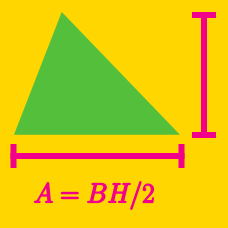Geometry

# Area of Triangles - Sine Rule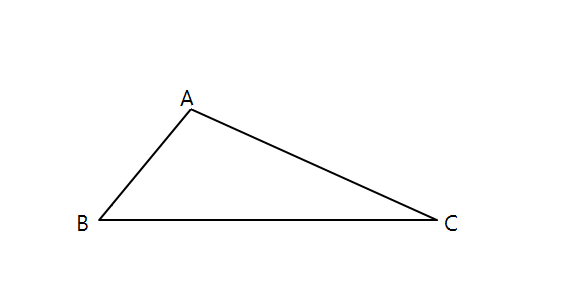In triangle $ABC,$ $AB=3,$ $AC = 12,$ and $\sin \angle BAC = 0.5.$ What is the area of triangle $ABC?$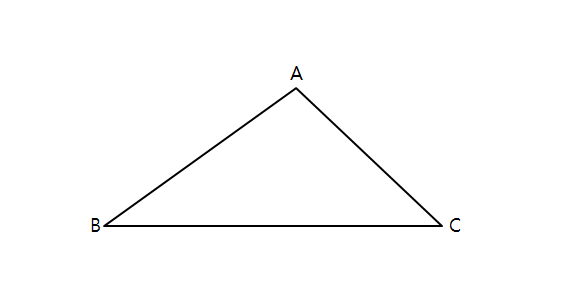In $\triangle ABC,$ $AC = 3$ and $\sin \angle ACB = \frac{2}{3}.$ If the area of $\triangle ABC$ is $9,$ what is $BC?$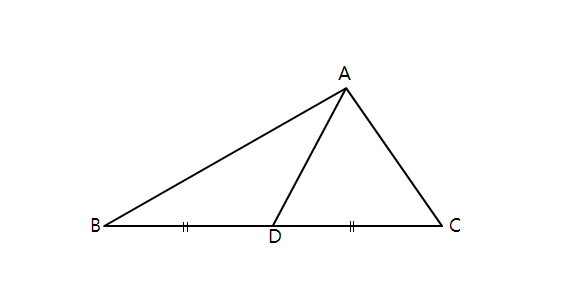In triangle $ABC$ above, $\overline{AD}$ bisects $\overline{BC}.$ Given that $AB = 12,$ $AD =8,$ and $\sin \angle BAD = \frac{1}{4},$ what is the area of $\triangle ABC?$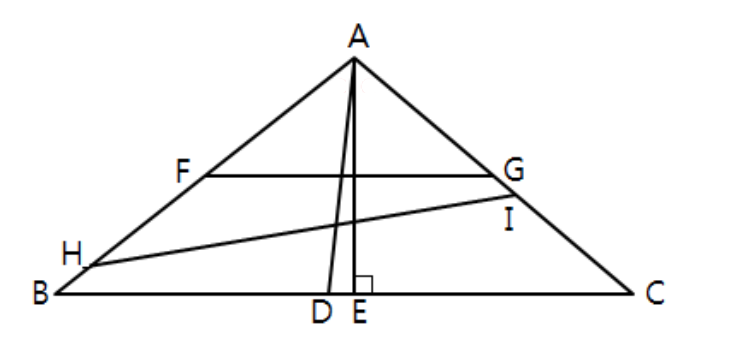In triangle $ABC,$ $\overline{AD}$ bisects $\angle BAC,$ $\overline{AE}$ is perpendicular to $\overline{BC},$ and $F$ and $G$ are the midpoints of $\overline{AB}$ and $\overline{AC},$ respectively. Given that $AB = 16,$ $AC=13,$ $AH=13,$ and $AI=8,$ and $\angle BAC = 90^{\circ},$ which of the following triangles has an area equal to half the area of $\triangle ABC?$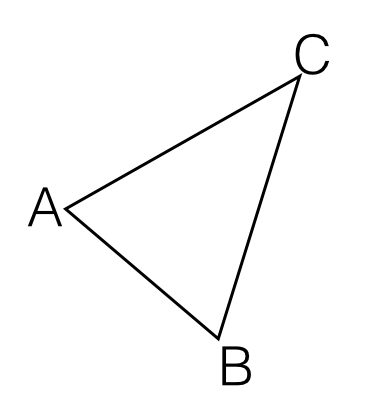In triangle $ABC,$ $AB = 3$ and $BC=6.$ If the area of the triangle is $6,$ what is $\sin \angle ABC?$

×